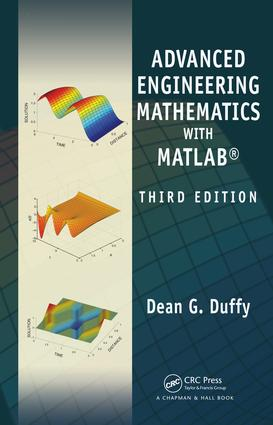# Advanced Engineering Mathematics with MATLAB

## 3rd Edition

CRC Press

1,105 pages

eBook (VitalSource) : 9780429184444
pub: 2010-10-26
from \$28.98

FREE Standard Shipping!

### Description

Taking a practical approach to the subject, Advanced Engineering Mathematics with MATLAB, Third Edition continues to integrate technology into the conventional topics of engineering mathematics. The author employs MATLAB to reinforce concepts and solve problems that require heavy computation. MATLAB scripts are available for download at www.crcpres

Introduction. Complex Variables. First-Order Ordinary Differential Equations. Higher-Order Ordinary Differential Equations. Fourier Series. The Fourier Transform. The Laplace Transform. The Z-Transform. The Hilbert Transform. The Sturm-Liouville Problem. The Wave Equation. The Heat Equation. Laplace's Equation. Green's Functions. Vector Analysis. Linear Algebra. Probability. Random Processes. Answers to the Odd-Numbered Problems. Index.

Dean G. Duffy is a former instructor at the US Naval Academy and US Military Academy. From 1980 to 2005, he worked on numerical weather prediction, oceanic wave modeling, and dynamical meteorology at NASA's Goddard Space Flight Center. Prior to this, he was a numerical weather prediction officer in the US Air Force from September 1975 to December 1979. He earned his Ph.D. in meteorology from MIT. Dr. Duffy has written several books on transform methods, engineering mathematics, Green's functions, and mixed boundary value problems.